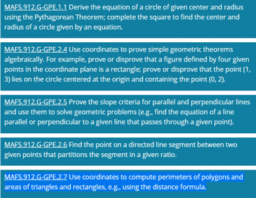# Expressing Geometric Properties with Equations (GPE) Cluster

Topic:
Equations
MAFS.912.G-GPE.1.1 Derive the equation of a circle of given center and radius using the Pythagorean Theorem; complete the square to find the center and radius of a circle given by an equation. MAFS.912.G-GPE.2.4 Use coordinates to prove simple geometric theorems algebraically. For example, prove or disprove that a figure defined by four given points in the coordinate plane is a rectangle; prove or disprove that the point (1, 3) lies on the circle centered at the origin and containing the point (0, 2). MAFS.912.G-GPE.2.5 Prove the slope criteria for parallel and perpendicular lines and use them to solve geometric problems (e.g., find the equation of a line parallel or perpendicular to a given line that passes through a given point). MAFS.912.G-GPE.2.6 Find the point on a directed line segment between two given points that partitions the segment in a given ratio. MAFS.912.G-GPE.2.7 Use coordinates to compute perimeters of polygons and areas of triangles and rectangles, e.g., using the distance formula.